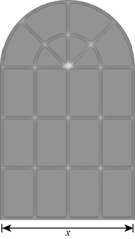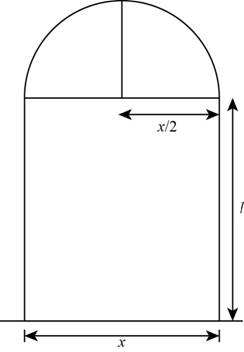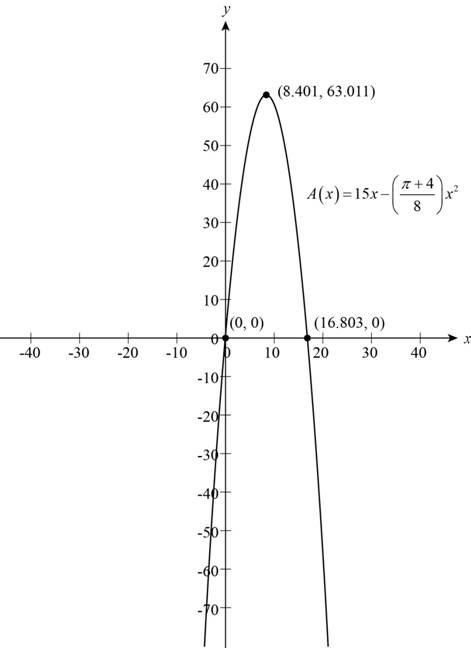# The function that models the area of the window.### Precalculus: Mathematics for Calcu...

6th Edition
Stewart + 5 others
Publisher: Cengage Learning
ISBN: 9780840068071### Precalculus: Mathematics for Calcu...

6th Edition
Stewart + 5 others
Publisher: Cengage Learning
ISBN: 9780840068071

#### Solutions

Chapter 2, Problem 25P

(a)

To determine

## To find: The function that models the area of the window.

Expert Solution

The function that models the area of the window is A(x)=15x(π+48)x2 .

### Explanation of Solution

Given:

A Norman window is in the shape of the rectangle with perimeter 30ft shown below.Figure (1)

Formula used:

The area of the rectangle is,

Perimeter of the rectangle is,

The area of the semi circle is,

Areaofsemicircle=πr22 (3)

Perimeter of the semi circle is,

Perimeterofsemi circle=πr (4)

Calculation:

Let the length in the Norman window is l units and the radius of the semicircle is r units.

The Norman window with the radius of the semicircle and the length of the rectangle is shown below.Figure (2)

The radius of the semicircle is,

r=x2

The perimeter of the Norman window is,

Perimeter of the Norman window=Perimeter of semicircle+Perimeterofrectangle 30=πr+x+2l

Substitute x2 for r in the above equation,

30=πx2+x+2l30=πx+2x+4l260=πx+2x+4l4l=60x(π+2)

Further solve the equation,

l=604x(π+2)4=15x(π+2)4

The length of the window is l=15x(π+2)4 .

The area of the Norman window is,

Area of  Norman window=Area of semicircle+AreaofrectangleA=πr22+lx

Substitute x2 for r and 15x(π+2)4 for l in the above equation,

A=π(x2)22+(15x(π+2)4)x=πx28+15xx2(π+2)4=15x+πx22x2π4x28=15x+x2π4x28

Further solve the above equation,

A(x)=15x+x2π4x28=15x(π+4)x28

Thus, the function that models the area of the window is A(x)=15x(π+48)x2 .

(b)

To determine

### To find: The dimension of the window that admits the greatest light.

Expert Solution

The width of the Norman window is 8.4ft and the length of the Norman window is 4.200ft .

### Explanation of Solution

From the part(a), the function that models the area of the window is A(x)=15x(π+48)x2 .

To find the dimension that admits the greatest amount of light, it needs to draw the graph of the function A(x)=15x(π+48)x2 .

The function contains the variable x is the breadth of the rectangular portion of the window.

The local maximum value of the function is the maximum finite value where the value of the function at the any number is greater than to the original function.

The condition for local minimum is,

f(a)f(x)

The graph of the function is shown below,Figure (3)

From the graph of the function shown as Figure (3) the greatest peak of the graph at the point (8.401,63.011) .

Then the maximum light admit from the Norman window is 63.011 at x=8.401 .

Substitute 8.4 for x in the length l=15x(π+2)4 ,

l=158.4(227+2)4=152.1(3.14+2)=152.15.14=1510.794=4.2

The length of the window is 4.200ft .

Thus, the width of the Norman window is 8.4ft and the length of the Norman window is 4.200ft .

### Have a homework question?

Subscribe to bartleby learn! Ask subject matter experts 30 homework questions each month. Plus, you’ll have access to millions of step-by-step textbook answers!